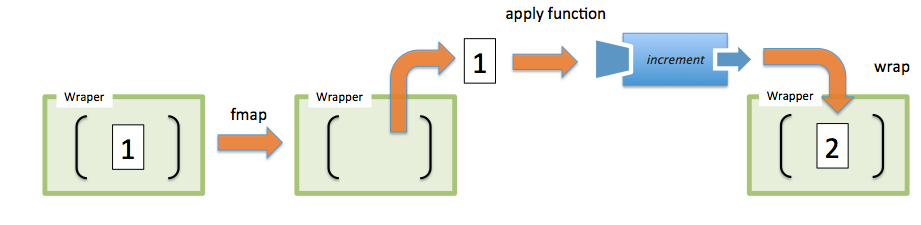# JavaScript Functors Explained

### A functor is nothing more than a data structure you can map functions over. Understand the JavaScript function.

· Web Dev Zone · Tutorial
Save
7.94K Views

In essence, a functor is nothing more than a data structure you can map functions over with the purpose of lifting values from a container, modifying them, and then putting them back into a container. Simply put, it is a design pattern that defines semantics for how fmap should work. Here’s the general definition of fmap:

``fmap :: (A -> B) -> Wrapper(A) -> Wrapper(B) ``

The function fmap takes a function (from A -> B) and a functor (wrapped context) Wrapper(A) and returns a new functor Wrapper(B) containing the result of applying said function onto the value and then closes it once more. Here’s a quick example using the increment function as our mapping function from A -> B (except in this case A and B are the same types):Figure 1 A value of 1 is contained within a container W, the functor is called with said wrapper and the increment function, which transforms the value internally and closes it back into a container.

Notice that because fmap basically returns a new copy of the container at each invocation, it can be considered to be immutable.

### Functor theory

A discussion on functors can easily get very formal and theoretical. If you do a quick web search for functors, you will find articles that will bombard you with terms such as: morphism and categories. The reason for this is that, like all functional programming techniques, functors originate from mathematics—in this case, category theory.

Without getting into the weeds, I can explain the basic meaning of this. Functors are defined as: “morphisms between categories.” All this really means is that a functor is an entity that defines the behavior of (fmap) that, given a value and function (morphism), maps said function onto a value of certain type (category) and generates a new functor.

Indeed, this is a bit theoretical to understand. Let’s go over a very simple example. Consider a simple 2 + 3 = 5 addition using functors. I can curry a simple add function to create a plus3 function as such:

``var plus = R.curry((a, b) => a + b);  var plus3 = plus(3);``

Now I will store the number two into a simple Wrapper functor:

``var two = wrap(2);``

Calling fmap to map plus3 over the container performs addition:

``````var five = two.fmap(plus3); //-> Wrapper(5)

five.map(R.identity); //-> 5``````

The outcome of fmap yields another context of the same type, which I can map R.identity over to extract its value. Notice that, because the value never escapes the wrapper, I can map as many functions as I want onto it and transform its value at every step of the way:

``two.fmap(plus3).fmap(plus10); //-> Wrapper(15)``

This can tricky to understand, so here’s is a visual of how fmap works again with plus3 in this figure:Figure 2 The value 2 has been added to a Wrapper container. The functor is used to manipulate this value, by first unwrapping it from the context, applying the given function onto it, and re-wrapping the value back into a new context.

The purpose of having fmap return the same type (or wrap the result again into a container) is so that we can continue chaining operations. Consider the following example that maps plus on a wrapped value and logs the result as shown in the following code:

``````var two = wrap(2);

two.fmap(plus3).fmap(R.tap(infoLogger)); //-> Wrapper(5)``````

Running this code prints the following message on the console:

InfoLogger [INFO] 5

Does this idea of chaining functions sound familiar? Actually, you’ve been using functors all along without realizing it. This is exactly what the map and filter functions do for arrays:

``````map    :: (A -> B)   -> Array(A) -> Array(B)

filter :: (A -> Boolean) -> Array(A) -> Array(A)``````

Functions map and filter are “homomorphism between categories.” The reason being is that both functions preserve the same type:

• homo: same

• morphism: a function that maintain structure

• category: type of value contained

Extending this concept into functions, consider another type of a homomorphic functor you’ve seen all along: compose. As you may know, the compose function is a mapping from functions into other functions:

``compose :: (B -> C) -> (A -> B) -> (A -> C)``

Functors, like any other functional programming artifact, are governed by some important properties:

They must be side effect free: mapping the R.identity function can be used to obtain the same value over a context. This proofs they are side effect free and preserves the structure of the wrapped value.

``wrap('Get Functional').fmap(R.identity); //-> Wrapper('Get Functional')``

They must be composable: this property indicates the composition of a function applied to fmap should be exactly the same as chaining fmap functions together. As a result, the following expression is exactly equivalent to the program previously:

``two.fmap(R.compose(plus3, R.tap(infoLogger))).map(R.identity); //-> 5``

Structures such as functors are prohibited from throwing exceptions, mutating elements on a list, or altering a function’s behavior. Their practical purpose is to create a context that allows you to securely manipulate and apply operations to values, without changing the original value. This is evident in the way map transforms one array into another without altering the original array; this concept equally translates to any container type.

However, functors by themselves aren’t too compelling and would fail in the presence of null data, just like the array map functor that effectively skips null elements and compose, which will skip invoking a null function object. This is analogous to having an empty catch block to ignore the failure. In practice, however, you will need to properly handle errors and for this you would need a new functional data type called Monads. You can learn more about functors, and Monads, in my book Functional Programming in JavaScript.